# Solving Linear Equations Linear Equations Equations like yx5

• Slides: 20Solving Linear Equations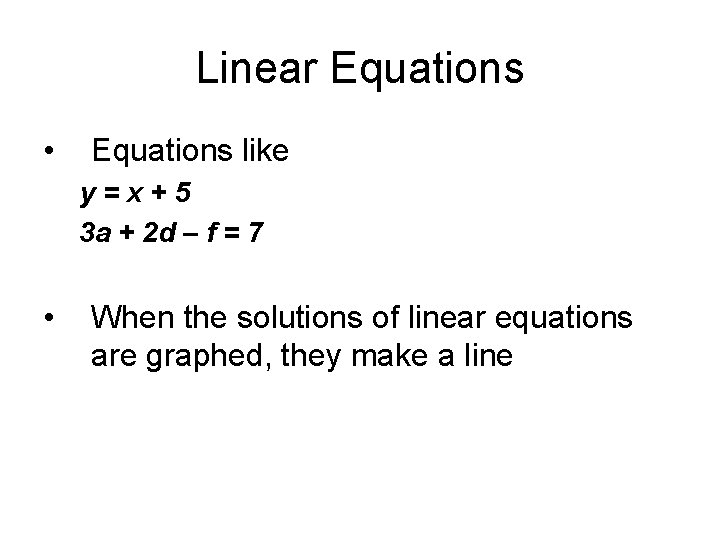Linear Equations • Equations like y=x+5 3 a + 2 d – f = 7 • When the solutions of linear equations are graphed, they make a line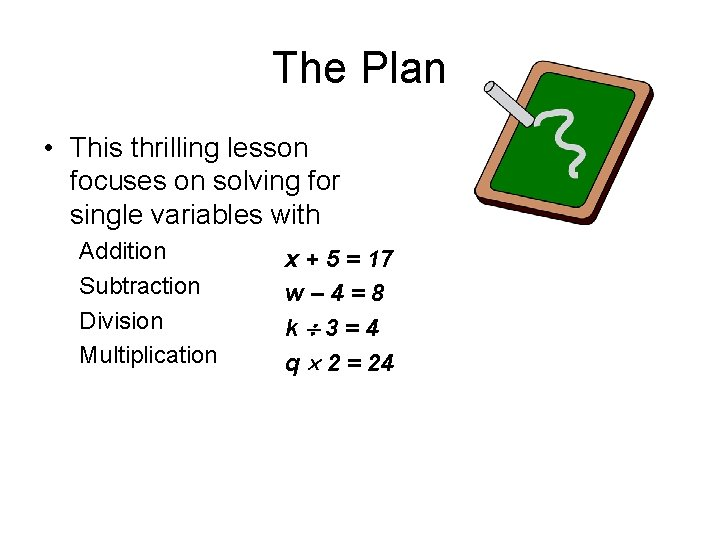The Plan • This thrilling lesson focuses on solving for single variables with Addition Subtraction Division Multiplication x + 5 = 17 w– 4=8 k 3=4 q 2 = 24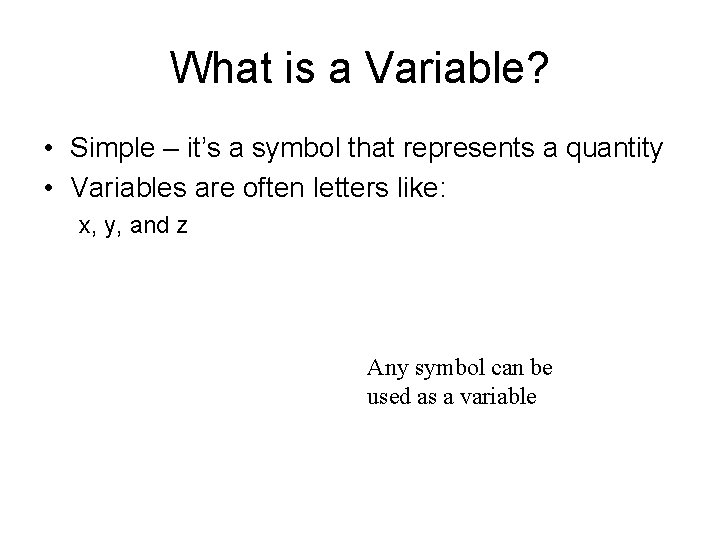What is a Variable? • Simple – it’s a symbol that represents a quantity • Variables are often letters like: x, y, and z Any symbol can be used as a variable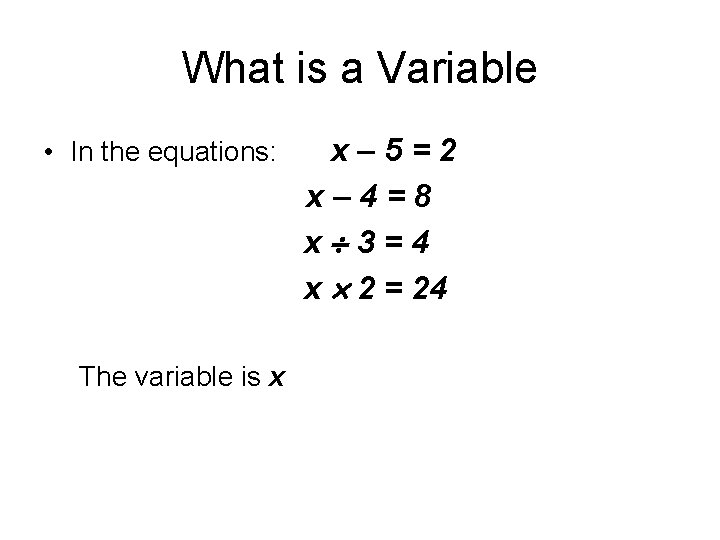What is a Variable • In the equations: The variable is x x– 5=2 x– 4=8 x 3=4 x 2 = 24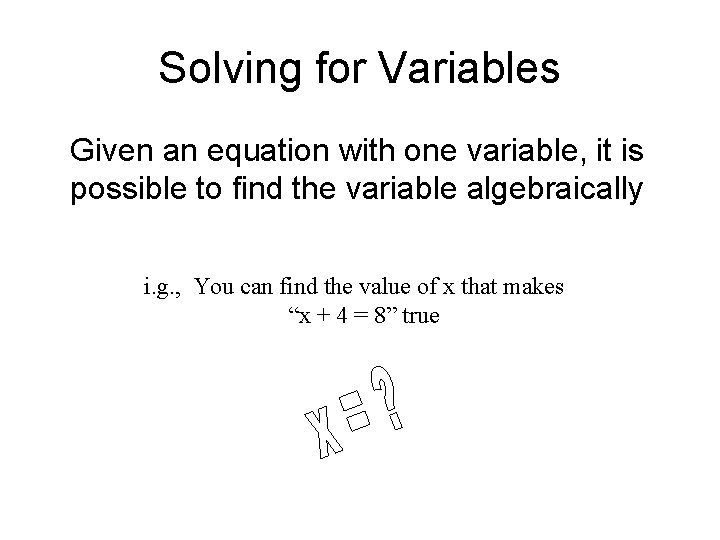Solving for Variables Given an equation with one variable, it is possible to find the variable algebraically i. g. , You can find the value of x that makes “x + 4 = 8” true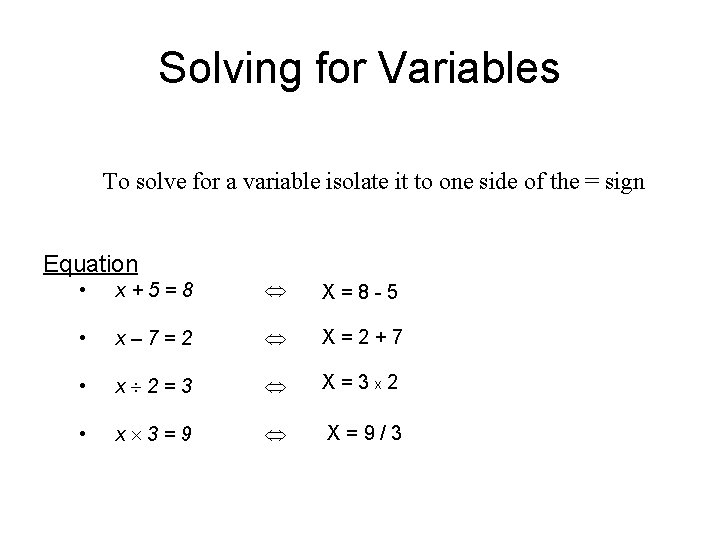Solving for Variables To solve for a variable isolate it to one side of the = sign Equation • x+5=8 X=8 -5 • x– 7=2 X=2+7 • x 2=3 X=3 x 2 • x 3=9 X=9/3Noticing Patterns Isolating a variable is easy…if you know how Do you notice any patterns between these equations and the previous? Equation Isolated Variable Equation • x+3=8 x=8– 3 • x– 5=2 x=2+5 • x 2=8 x=8 2 • x 3 = 12 x = 12 3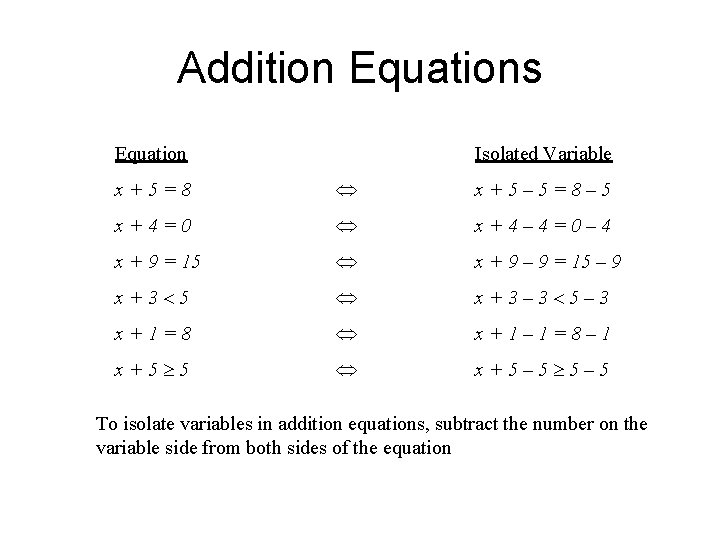Addition Equations Equation Isolated Variable x+5=8 x+5– 5=8– 5 x+4=0 x+4– 4=0– 4 x + 9 = 15 x + 9 – 9 = 15 – 9 x+3 5 x+3– 3 5– 3 x+1=8 x+1– 1=8– 1 x+5 5 x+5– 5 To isolate variables in addition equations, subtract the number on the variable side from both sides of the equation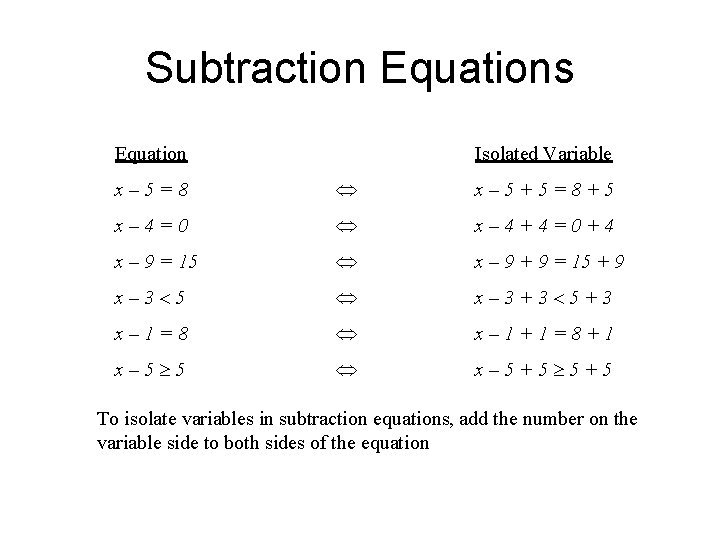Subtraction Equations Equation Isolated Variable x– 5=8 x– 5+5=8+5 x– 4=0 x– 4+4=0+4 x – 9 = 15 x – 9 + 9 = 15 + 9 x– 3 5 x– 3+3 5+3 x– 1=8 x– 1+1=8+1 x– 5 5 x– 5+5 To isolate variables in subtraction equations, add the number on the variable side to both sides of the equationDivision Equations Equation Isolated Variable x 5 = 15 x 5 5 = 15 5 x 4 = 16 x 4 4 = 16 4 x 9=0 x 9 9=0 9 x 3 6 x 3 3 6 3 x 1 8 x 1 1 8 1 x 5=5 x 5 5=5 5 To isolate variables in multiplication equations, divide the number on the variable side from both sides of the equation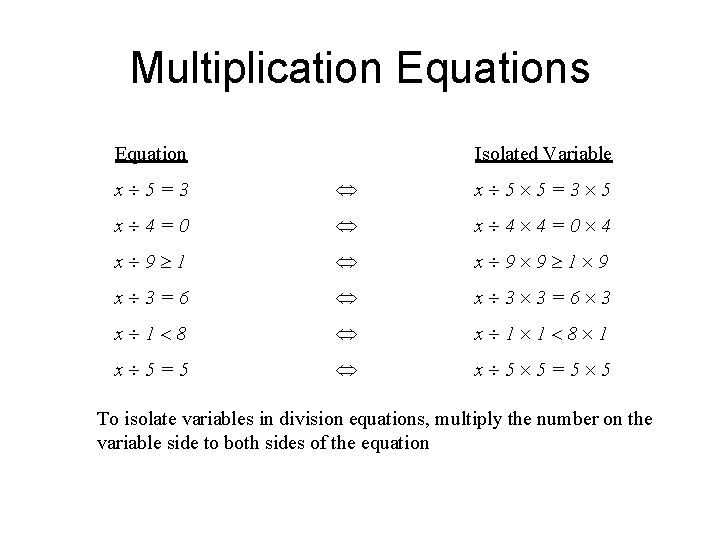Multiplication Equations Equation Isolated Variable x 5=3 x 5 5=3 5 x 4=0 x 4 4=0 4 x 9 1 x 9 9 1 9 x 3=6 x 3 3=6 3 x 1 8 x 1 1 8 1 x 5=5 x 5 5=5 5 To isolate variables in division equations, multiply the number on the variable side to both sides of the equation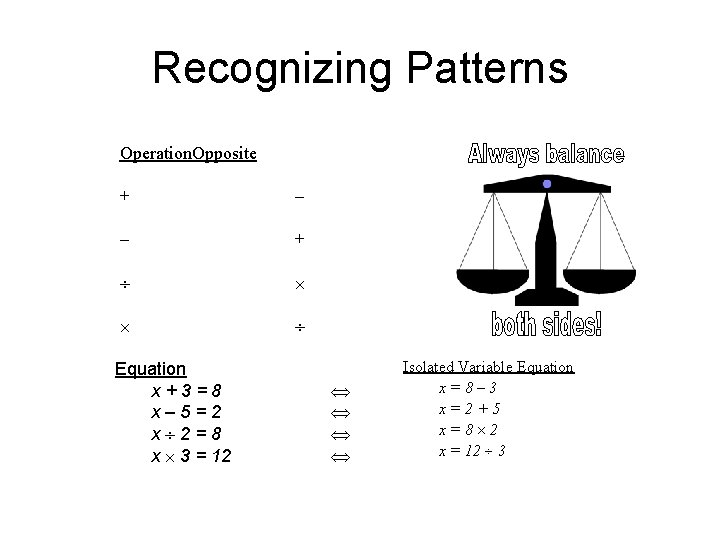Recognizing Patterns Operation. Opposite + – – + Equation x+3=8 x– 5=2 x 2=8 x 3 = 12 Isolated Variable Equation x=8– 3 x=2+5 x=8 2 x = 12 3Recognizing Patterns • Use the opposite of an operation with the number to isolate the variable Operation Opposite + – – + Equation x+3=8 x– 5=2 x 2=8 x 3 = 12 Isolated Variable Equation x=8– 3 x=2+5 x=8 2 x = 12 3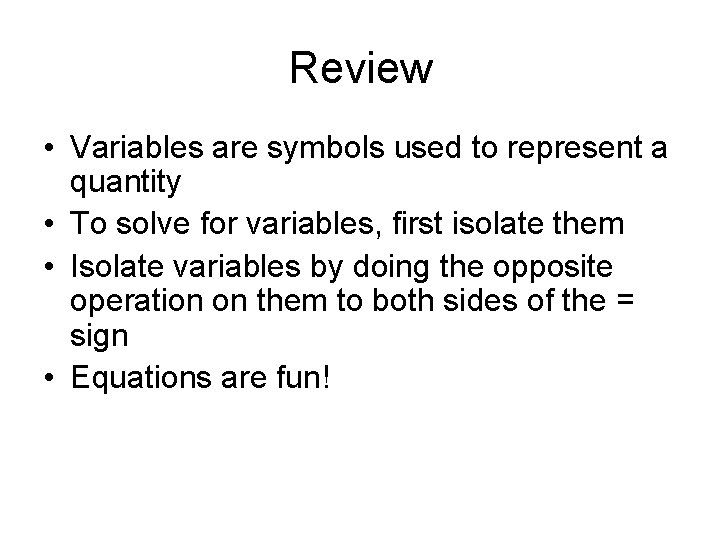Review • Variables are symbols used to represent a quantity • To solve for variables, first isolate them • Isolate variables by doing the opposite operation on them to both sides of the = sign • Equations are fun!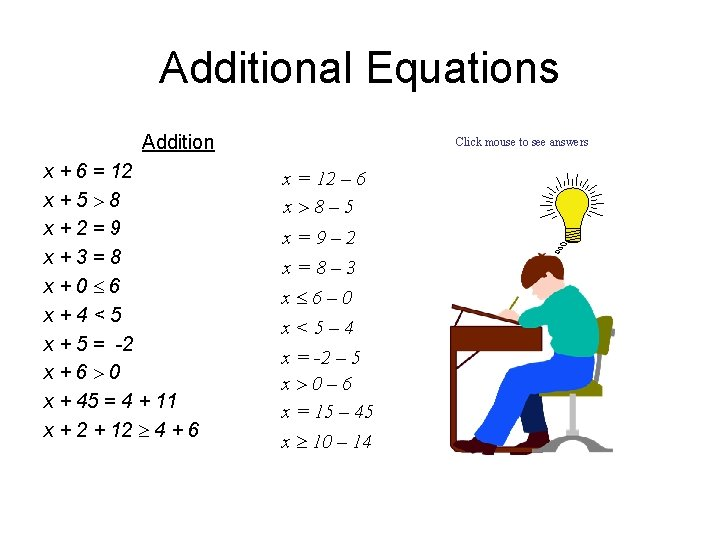Additional Equations Addition x + 6 = 12 x+5 8 x+2=9 x+3=8 x+0 6 x+4<5 x + 5 = -2 x+6 0 x + 45 = 4 + 11 x + 2 + 12 4 + 6 Click mouse to see answers x = 12 – 6 x 8– 5 x=9– 2 x=8– 3 x 6– 0 x<5– 4 x = -2 – 5 x 0– 6 x = 15 – 45 x 10 – 14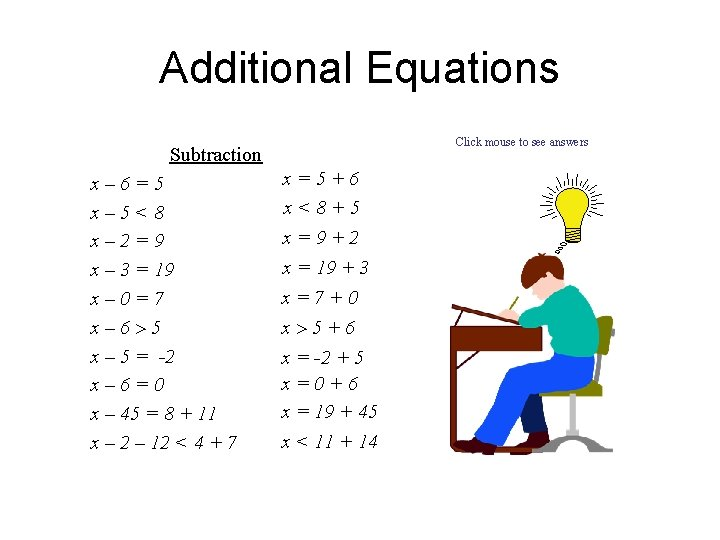Additional Equations Click mouse to see answers Subtraction x– 6=5 x– 5<8 x– 2=9 x – 3 = 19 x– 0=7 x– 6 5 x – 5 = -2 x– 6=0 x – 45 = 8 + 11 x – 2 – 12 < 4 + 7 x=5+6 x<8+5 x=9+2 x = 19 + 3 x=7+0 x 5+6 x = -2 + 5 x=0+6 x = 19 + 45 x < 11 + 14Additional Equations Division x 6 = 12 x 5 = 10 x 2 = 16 x 3<3 x 1=6 x 4 = 20 x 5 -3 x 6=0 x 3 = 15 x 2 10 Click mouse to see answers x = 12 6 x = 10 5 x = 16 2 x<3 3 x=6 1 x = 20 4 x -3 5 x=0 6 x = 15 3 x 10 2Additional Equations Multiplication x 6 = 12 x 5 10 x 2 16 x 3=3 x 1=6 x 4 < 20 x 5 = -15 x 6=0 x 3 = 15 x 2 10 Click mouse to see answers x = 12 6 x 10 5 x 16 2 x=3 3 x=6 1 x < 20 4 x = -15 5 x=0 6 x = 15 3 x 10 2THE END